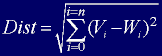Learning Algorithm Overview

A SOM does not need a target output to be specified unlike many other types of network. Instead, where the node weights match the input vector, that area of the lattice is selectively optimized to more closely resemble the data for the class the input vector is a member of. From an initial distribution of random weights, and over many iterations, the SOM eventually settles into a map of stable zones. Each zone is effectively a feature classifier, so you can think of the graphical output as a type of feature map of the input space. If you take another look at the trained network shown in  figure 1, the blocks of similar colour represent  the individual zones. Any new, previously unseen input vectors presented to the network will stimulate nodes in the zone with similar weight vectors.

Training occurs in several steps and over many iterations:

1. Each node's weights are initialized.

2. A vector is chosen at random from the set of training data and presented to the lattice.

3. Every node is examined to calculate which one's weights are most like the input vector. The winning node is commonly known as the Best Matching Unit (BMU).

4. The radius of the neighbourhood of the BMU is now calculated. This is a value that starts large, typically set to the 'radius' of the lattice,  but diminishes each time-step. Any nodes found within this radius are deemed to be inside the BMU's neighbourhood.

5. Each neighbouring node's (the nodes found in step 4) weights are adjusted to make them more like the input vector. The closer a node is to the BMU, the more its weights get altered.

6. Repeat step 2 for N iterations.

The Learning Algorithm In Detail

Now let's take a look at each step in detail. I'll supplement my descriptions with extracts from the source code where appropriate. Hopefully, for those of you who can program, the code will help reinforce your understanding of the principles involved.

Initializing The Weights

Prior to training, each node's weights must be initialized. Typically these will be set to small standardized random values. The weights in the SOM from the accompanying code project are initialized so that 0 < w < 1.  Nodes are defined in the source code by the class CNode. Here are the relevant parts of that class:

 class CNode { private:   //this node's weights   vector      m_dWeights;     //its position within the lattice   double              m_dX,                       m_dY;     //the edges of this node's cell. Each node, when draw to the client   //area, is represented as a rectangular cell. The colour of the cell   //is set to the RGB value its weights represent.   int                 m_iLeft;   int                 m_iTop;   int                 m_iRight;   int                 m_iBottom;     public:   CNode(int lft, int rgt, int top, int bot, int NumWeights):m_iLeft(lft),                                                             m_iRight(rgt),                                                             m_iBottom(bot),                                                             m_iTop(top)   {     //initialize the weights to small random variables     for (int w=0; w

You can see that the weights are initialized automatically by the constructor when a CNode object is instantiated. The member variables m_iLeft, m_iRight, m_iTop and m_iBottom are only used to render the network to the display area - each node is represented by a rectangular cell described by these values, as shown previously in Figure 3.

Calculating the Best Matching Unit

To determine the best matching unit, one method is to iterate through all the nodes and calculate the Euclidean distance between each node's weight vector and the current input vector. The node with a weight vector closest to the input vector is tagged as the BMU.

The Euclidean distance is given as:Equation 1

where V is the current input vector and W is the node's weight vector. In code this equation translates to:

 double CNode::GetDistance(const vector &InputVector) {   double distance = 0;     for (int i=0; i

As an example, to calculate the distance between the vector for the colour red (1, 0, 0) with an arbitrary weight vector (0.1, 0.4, 0.5)

distance = sqrt( (1 - 0.1)2 + (0 - 0.4)2+ (0 - 0.5)2 )

= sqrt( (0.9)2 + (-0.4)2+ (-0.5)2 )

= sqrt( 0.81 + 0.16+ 0.25 )

= sqrt(1.22)

distance  = 1.106

1   2   3   4   5   Home